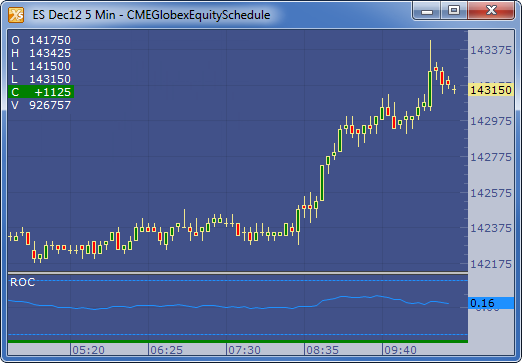← Back to X_TRADER® Help Library

### X_STUDY® Documentation

#### Rate of Change (ROC)

##### Description

The Rate of Change (ROC) indicator compares the current price with the previous price from a selected number of periods ago. The current price is divided by the previous price and expressed as a percentage. This indicator is also commonly known as a momentum indicator.

##### Formula

ROC = (Current Price / Price of n bars ago)-1.0) * 100

Where: n = Time period

##### Example